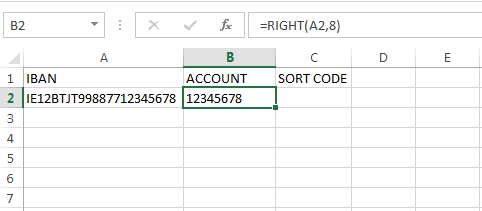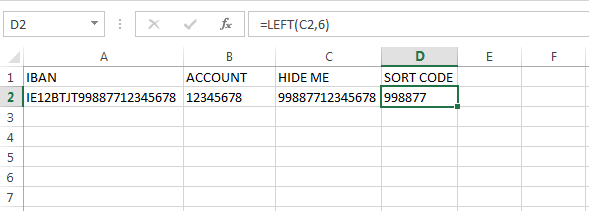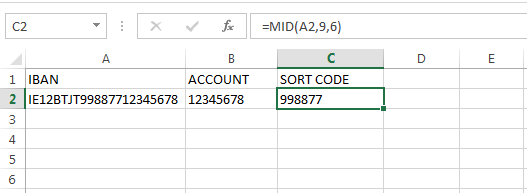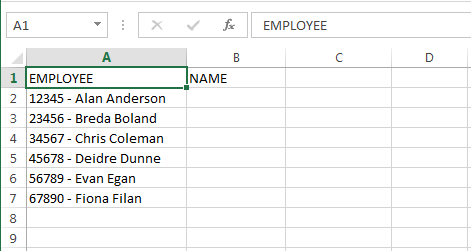# THE LEN LEFT RIGHT MID FORMULAS IN EXCELThe LEN LEFT RIGHT MID Formula are 4 Excel formula that can be really useful.  They might not be used that often, but when you need them, they can save you a lot of time.

I will go through each of the 4 formulas and then give examples of where they can be combined.

For the LEN, LEFT, and RIGHT formula, I will use an example of bank account details to demonstrate the formula.  At the minute (2014) there are changes in Europe regarding standardizing bank details.

For us here in Ireland, this means that our old 8 digit account number will become a 22-digit IBAN (International Bank Account).  This is made up of 2 letters for the country, 2 numbers and then 4 letters for the bank, the old 6-digit sort code and then the old 8-digit bank account number.

Why am I going through this with you?  Obviously this isn’t a post about banking changes, what I am showing you here will be some formula that I have shared with a few people who wanted to compare the new IBAN details against the old bank details.  Using LEN, LEFT and RIGHT this can easily be done.

So, for demonstration here, the old bank account details and IBAN are

• Country : Ireland
• Bank : The JayTray Bank
• Sort Code : 99-88-77
• Account : 12345678
• IBAN : IE12BTJT99887712345678

Obviously these are made up bank details!!

## RIGHT

The RIGHT formula returns the rightmost characters from a string.

The syntax is =RIGHT(Text,[num_chars]).  Text is the string that we want to query and num_chars is the number of characters we want to return.

If our num_chars was 1, this would return the last character (rightmost), if the num_chars was 2, this would return the last 2 characters.

Text can be text typed into the formula, or cell reference.

If we did =RIGHT(“Hello”, 3) our result would be “llo” the rightmost 3 characters in our string.

If we typed =RIGHT(A2,8) then our result would give us the rightmost 8 characters in the value of cell A2.

In our case, we want the last 8 digits of the IBAN which is our old account number.  If our IBAN is in A2, then the formula is =RIGHT(A2,8).## LEFT

Like the RIGHT formula, the LEFT formula returns a number of characters from a string, this time the leftmost characters.

The syntax is =LEFT(Text,[num_chars]).  Text is the string that we want to query and num_chars is the number of characters we want to return.

If our num_chars was 1, this would return the first character (leftmost), if the num_chars was 2, this would return the first 2 characters.

Text can be text typed into the formula, or cell reference.

If we did =LEFT(“Hello”, 3) our result would be “Hel” the leftmost 3 characters in our string.

If we typed =LEFT(A2,8) then our result would give us the leftmost 8 characters in the value of cell A2.

In our example, we are going to use the LEFT formula to get our sort code.  The 6-digit sort code is in the middle of our IBAN, how can we pull it out using the LEFT formula?

If we typed =LEFT(A2, 6) we would get “IE12BT”, the 6 leftmost characters, but not our sort code.

By using the RIGHT and LEFT formula we can get the sort code.  The rightmost 14 characters gives us our sort code and account number, of this, the first 6 characters gives us our sort code.

We can do this two ways, one would be to do the right formula in column C in our example (and hide that column later) and then use our LEFT formula to get the first 6 characters from column C.Another way to do this would be to combine the RIGHT and LEFT formula in one, as below.What does this formula mean?  Really, it is no different to our previous example where we used the RIGHT formula to get the last 14 characters and then the LEFT formula to get the first 6 digits of this.  It may look more complicated, but it’s not that different if you breakdown the formula.  Read this post on brackets and commas for more on this.

I’ll breakdown the formula:

• =LEFT( : Overall, our formula is a LEFT formula we are going to get the first 6 characters from a string
• RIGHT(A2,14) : Our text that we are going to query is the result of the RIGHT formula which is the last 14 characters from cell A2
• 6) : The number of characters from our LEFT statement is 6.  Note the number of characters from the RIGHT formula were contained within that formula’s brackets.

But if this is confusing you, fear not, the MID formula can do the same thing.

## MID

The MID formula returns a number of characters after a starting position from the middle of a string of text.

The syntax is =MID(Text, start_num, num_chars).

Text again, is the text or cell reference that we want to query.

start_num is the starting position that we are going to retrieve text from.  It is a numerical value.  In our case, the first 8 characters relate to the country and bank.  The 9th character is the start of our sort code, so our starting position is 9.

num_chars again is the number of characters that we want to return, the sort code is 6 characters long.

Our formula is =MID(A2,9,6).Querying cell A2, we want the 6 characters starting from the 9th.

## LEN

The LEN formula returns the length, or number of characters in a string.

The syntax is =LEN(Text).  The number of characters that makes up the length of a string includes spaces and punctuation.

In cell A2, if I type “Hello, how are you?”, this is made up of 14 letters, but 19 characters including the spaces, comma and question mark.

The LEN formula here is =LEN(A2) and this returns the value of 19.You could also type the text into your formula and get the same result =LEN(“Hello, how are you?”).

You’re probably wondering by now why would I ever need to know this formula?

One example of where I was asked for help and I used the LEN formula was when a report listed employee names and numbers together, like 12345 – Alan Anderson.  The user only wanted the name.I could use the RIGHT formula to return the last 13 characters which would give us “Alan Anderson” which is what we want, but the last 13 characters of the other employees might not be just the name, for example the last 13 characters of Evan is “9 – Evan Egan”.

How can we tell the formula to change the number of characters we want to retrieve??

With the LEN formula!

We want to ignore the first 8 characters of each employee, this is the 5 digit number, 2 spaces and a hyphen.  We will use the LEN formula to calculate the number of characters for each employee and then deduct 8.  This result gives us the number of characters that we need for our RIGHT formula.

By using LEN(A2)-8 within our RIGHT formula we get the number of characters we need.  For Alan, this is 13, for Breda it’s 12, for Christ it’s 13, 9 for Evan, etc.

Instead of us having to enter the number of characters for each employee, we can use the LEN formula to count the number of characters and then deduct 8 from this number to give us the number of characters that we do want.Some of you out there may be wondering why not just use “Text to Columns” to separate the names from the numbers using the hyphen as a way to identify the gaps.  The answer is simply because some people in the list had hyphenated names which could cause problems here.

Or, using “Text to Columns” at fixed points we could separate the names from the numbers, but the user wanted a formula solution that they could use repeatedly with different files and types of text.

## Combining Formulas

As you have seen, combining formulas together can give you more choice in how you analyse data or tackle problems.

Formulas can be used within formulas too – you have also seen that you don’t have to select a number of characters for some of the formula, instead you can use a formula to calculate the number of characters.

When you start combining formulas like this, or if you are looking at formulas created by somebody else, remember to use the brackets and commas to breakdown the formula.